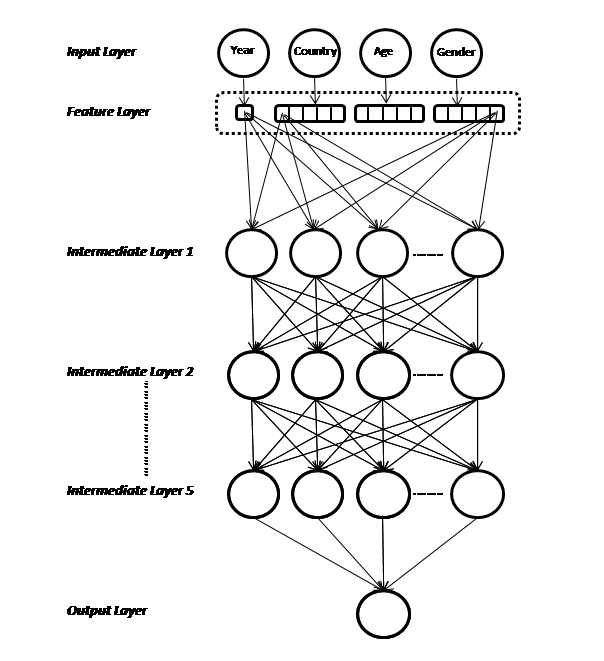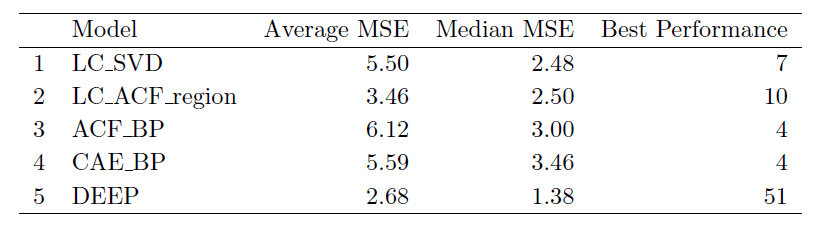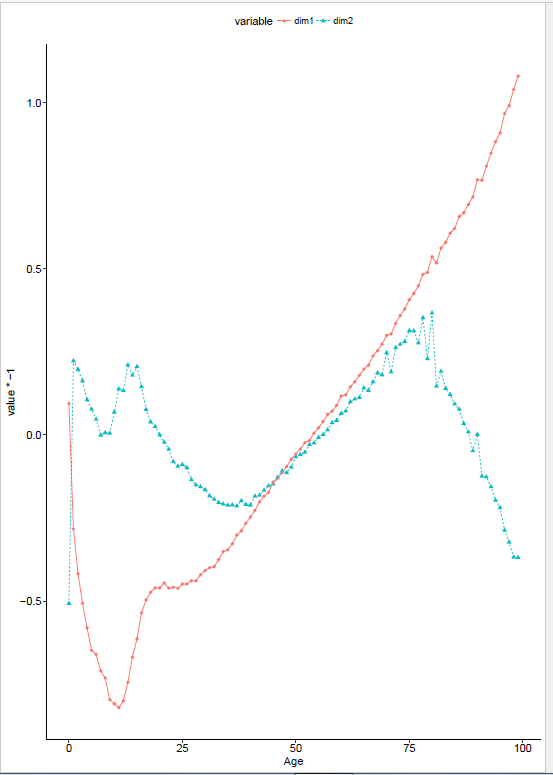# Neural networks, the Lee-Carter Model and Large Scale Mortality  Forecasting

This post discusses a new paper that I am very glad to have co-authored with Mario Wüthrich, in which we apply deep neural networks to mortality forecasting. The draft can be found here:

Paper

A topic that I have been interested in for a long time is forecasting mortality rates, perhaps because this is one of the interesting intersections of statistics and these days, machine learning, and the field of actuarial science in life insurance. Several methods to model mortality rates over time have been proposed, ranging from the relatively simple method of extrapolating mortality rates directly using time series, to more complicated statistical approaches.

One of the most famous of these is the Lee-Carter method, which models mortality as an average mortality rate that changes over time. The change over time is governed by a time-based mortality index, which is common to all ages, and an age-specific rate of change factor:

ln(mx,t )=ax +kt .bx

where mx,t is the force of mortality at age x in year t, ax is average log mortality rate during the period at age x, κt is the time index in year in year t and bx is the rate of change of log mortality with respect to the time index at age x.

How are these quantities derived? There are two methods prominent in the literature – applying Principal Components Analysis, or Generalized Non-linear Models, which are different from GLMs in the sense that the user can specify non-additive relationship between two or more terms. To forecast mortality, models are first fit to historical mortality data and the coefficients (in the case of the Lee- Carter model, the vector κ) are then forecast using a time series model, in a second step.

In the current age of big data, relatively high quality mortality data spanning an extended period are available for many countries from the excellent Human Mortality Database, which is a resource that anyone with an interest in the study of mortality can benefit from. Other interesting sources are databases containing sub-national rates for the USA, Japan and Canada. The challenge, though, is how to model all of these data simultaneously to improve mortality forecasts? While some extensions of basic models like the Lee-Carter model have been proposed, these rely on assumptions that might not necessarily be applicable in the case of large scale mortality forecasting. For example, some of the common multi-population mortality models rely on the assumption of a common mortality trend for all countries, which is likely not the case.

In the paper, we tackle this problem in a novel way – feed all the variables to a deep neural network and let it figure out how exactly to model the mortality rates over time. This speaks to the idea of representation learning that is central to modern deep learning, which is that many datasets, such as large collections of images as in the ImageNet dataset, are too complicated to model by hand-engineering features, or it is too time consuming to perform the modelling. Rather, the strategy in deep learning is to define a neural network architecture that expresses useful priors about the data, and allow the network to learn how the raw data relates to the problem at hand. In the example of modelling mortality rates, we use two architectural elements that are common in applications of neural networks to tabular data:

• We use a deep network, in other words the network consists of multiple layers of variables that expresses the prior that complex features can be represented by a hierarchy of simpler representations learned in the model.
• Instead of using one-hot encoding to signify to the network when we are modelling a particular country, or gender, we use embedding layers. When applied to many categories, one-hot encoding produces a high-dimensional feature vector that is sparse (i.e. many of the entries are zero), leading to potential difficulties in fitting a model as there might not be enough data to derive credible estimates for each category. Even if there is enough data, as in our case of mortality rates, each parameter is learned in isolation, and the estimated parameters do not share information, unless the modeller explicitly chooses to use something like credibility or mixed models. The insight of Bengio et al. (2003) to solve these problems is that categorical data can successfully be encoded into low dimensional, dense numerical vectors, so, for example, in our model, country is encoded into a five-dimensional vector.

In the paper, we also show how the original Lee-Carter model can be expressed as a neural network with embeddings!

Here is a picture of the network we have just described:In the paper, we also employ one of the most interesting techniques to emerge from the computer vision literature in the past several years. The original insight is due to the authors of the ResNet paper, who analysed the well-known problem that it is often difficult to train deep neural networks. They considered that a deep neural network should be no more difficult to train than a shallow network, since the deeper layers could simply learn the identity function, and thus be equivalent to a shallow network. Without going too far off track into these details, their solution is simple – add skip layers that connect the deep layers to more shallow layers in the network. This idea is expanded on in the DenseNet architectures. We simply added a connection between the feature layer, and the fifth layer of the network, connecting the embedding layers almost to the deepest layer of the network. This boosted the performance of the networks considerably.

We found that the deep neural networks dramatically outperformed the competing methods that we tested, forecasting with the lowest MSE in 51 out of the 76 instances we tested! Here is a table comparing the methods, and see the paper for more details:Lastly, an interesting property of the embedding layers learned by neural networks is the fact that the parameters of these layers are often interpretable as so-called “relativities” (to use some actuarial jargon), in other words, as defining the relationship between the different values that a variable may take.  Here is a picture of the age embedding, which shows that the main relationship learned by the network is the characteristic shape of a modern life table:This is a rather striking result, since at no time did we specify this to the network! Also, once the architecture was specified, the network has also learned to forecast mortality rates more successfully than human specified models reminding me of one of the desiderata for AI systems listed by Bengio (2009):

“Ability to learn with little human input the low-level, intermediate, and high-level abstractions that would be useful to represent the kind of complex functions needed for AI tasks.”

We would value any feedback on the paper you might have.

References

Bengio, Y. 2009. “Learning deep architectures for AI”, Foundations and trends® in Machine Learning 2(1):1-127.

This site uses Akismet to reduce spam. Learn how your comment data is processed.